Next: Integration Circuit Up: Mathematical Operations Previous: Current Summing Amplifier

### Differentiation Circuit

To obtain a differentiation circuit we replace the input resistor of the inverting amplifier with a capacitor as shown in figure 6.9.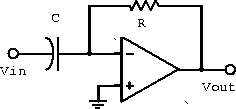Figure 6.9:  Differentiation circuit.

Replacing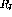with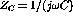in the voltage gain gives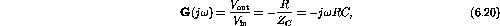or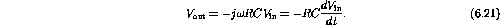Using dV/dt=I/C gives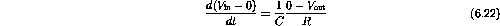and thus the same result as above.

The frequency response is shown in figure 6.10.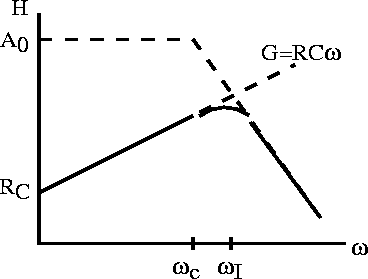Figure 6.10:  Differentiation circuit frequency response.

Doug Gingrich
Tue Jul 13 16:55:15 EDT 1999